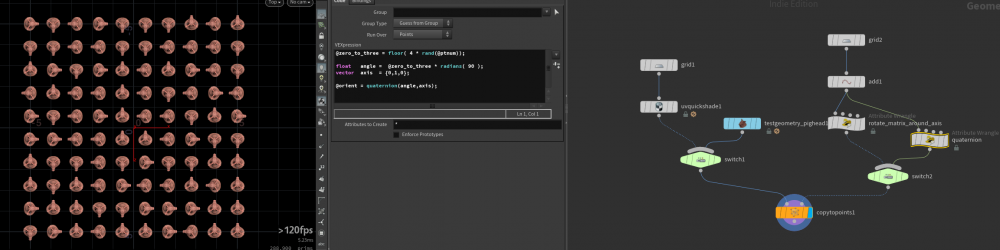## Recommended Posts

I copy the small grids to each point of the grid template and now I would like to rotate them in randomly so they snap to 90/180 and 270-degree intervals in Y-axis. Is there an easy way to solve it in VEX or with VOPS?##### Share on other sites

Hi Dominic,
here is one possible way. File is attached.

```@zero_to_three = floor( 4 * rand(@ptnum));

float   angle =  @zero_to_three * radians( 90 );
vector  axis  = {0,1,0};
matrix3 m     = ident();
rotate(m, angle, axis);

@up = {0,1,0};
@N  = m * {0,0,1};```
Edited by ikoon
•1

##### Share on other sites
2 minutes ago, ikoon said:

Hi Dominic,
here is one possible way. File is attached.

```
@zero_to_three = floor( 4 * rand(@ptnum));

float   angle =  @zero_to_three * radians( 90 );  // 45 in 0 to 90
vector  axis  = {0,1,0};
matrix3 m     = ident();
rotate(m, angle, axis);

@N = m * {0,0,1};```

Thats amazing, thank you.

##### Share on other sites

Here is the same result, but orientation is set by the @orient attribute:

```@zero_to_three = floor( 4 * rand(@ptnum));

float   angle =  @zero_to_three * radians( 90 );
vector  axis  = {0,1,0};

@orient = quaternion(angle,axis);```quaternion.hiplc

•3

##### Share on other sites
13 minutes ago, ikoon said:

Here is the same result, but orientation is set by the @orient attribute:

```
@zero_to_three = floor( 4 * rand(@ptnum));

float   angle =  @zero_to_three * radians( 90 );
vector  axis  = {0,1,0};

@orient = quaternion(angle,axis);```

much cleaner, thank you## Create an account

Register a new account